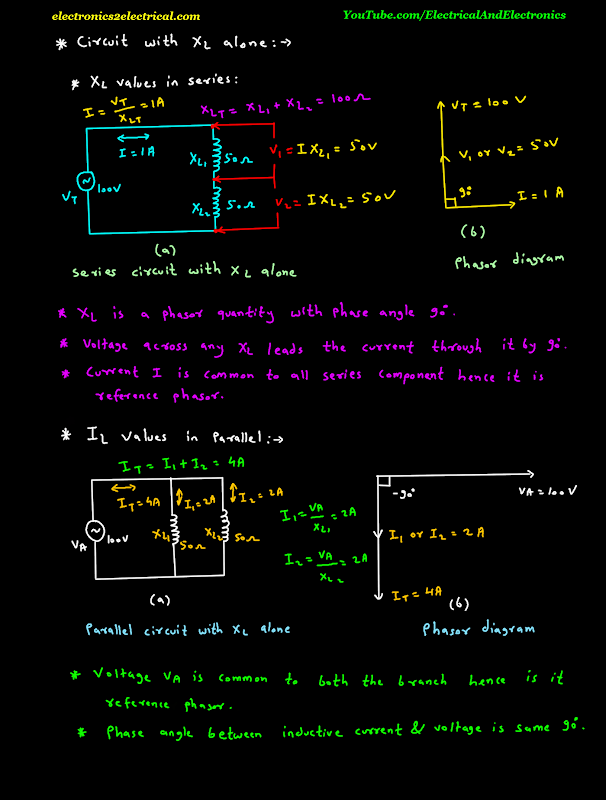Circuits with XL Alone

edited

0 like 0 dislike

Circuits with XL Alone

X L values in series

let two inductive reactances X L 1 and X L 2 are connected in series and has value 50 ohm and 50 ohm respectively.

100 volts A C voltage source is applied.

we can find series current flowing in the circuit by ohm's law.

So current I is equal to voltage V T upon total inductive reactance X L T.

Total inductive reactance X L T is equal to X L 1 plus X L 2.

Voltage across X L 1 is V 1 is equal to series current I into X L 1.

Voltage across X L 2 is V 2 is equal to series current I into X L 2.

Inductive reactance X L is a phasor quantity with phase angle 90 degree.

Voltage across any X L leads the current through it by 90 degree.

Current I is common to all series component hence it is reference phasor.Now inductive current I L values in parallel

Now Let two inductive reactances are connected in parallel and 100 volts A C source is applied.

The current flowing through inductive reactance 1 is I 1 is equal to V A upon X L 1.

The current flowing through inductive reactance 2 is I 2 is equal to V A upon X L 2.

So the total current is equal to current I 1 plus current I 2.

The figure b shows the phasor diagram.

Voltage V A is common to both the branch hence it is reference phasor.

And it is taken horizontal.

Phase angle between inductive current and voltage is same 90 degree.

edited